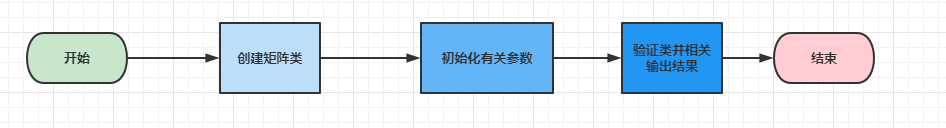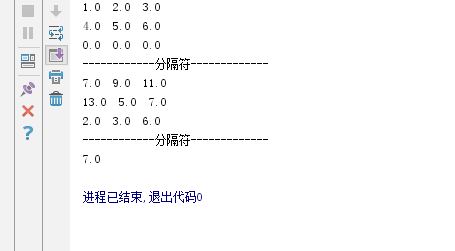# 【数据结构】利用 Python 手把手带你自定义矩阵

• 2022 年 9 月 22 日
广东
• 本文字数：3048 字

阅读完需：约 10 分钟

# 前言

## 什么是矩阵

，在计算机中，线性代数是非常重要的一门学科，计算机大部分计算都是利用的线性代数里面的矩阵运算，

# 自定义矩阵的前置知识

Python 将所有以两个下划线开头的类保留为魔术方法。所以在定义类方法时，除了上述的魔术方法，建议不要以下划线为前缀。

1. __new__(cls[,...]):在一个对象实例化的时候调用第一个方法.他的第一个参数是这个类,其他的参数直接传递给__init__()方法.__new__()方法决定是否使用__init__().因为__new__()可以调用其他类的构造方法或者直接返回其他实例对象作为本类实例.如果__new__()没有返回实例对象,则,__init__()方法不会被调用.__new__()主要是用于继承一个不可变的类型,如一个 tuple 或者 string.注:我们一般不会重写该方法

2. __init__(self[,...]):构造器或者构造方法,相当于 java 和 PHP 中的构造函数,初始化类的时候被调用,用于对数据的初始化

3. __del__(self):析构器或者析构方法,相当于 php 中的析构函数.当实例化对象被彻底销毁时被调用（实例化对象的所有指针都被销毁时被调用）

4. __getitem__(self,key):定义获取容器中指定元素的行为，相当于 self[key]

5. __setitem__(self, key, value):定义设置容器中指定元素的行为，相当于 self[key] = value

6. __delitem__(self, key):定义删除容器中指定元素的行为，相当于 del self[key]

7. __eq__(self, other):这个是比较操作符,定义等于号的行为：x == y 调用 x.__eq__(y)

9. __sub__(self, other):这个是算术运算符,定义减法的行为：-

10. __mul__(self, other):定义乘法的行为：*

11. __truediv__(self, other):定义真除法的行为：/

12. __len__(self):定义当被 len() 调用时的行为（一般返回容器类的长度）

# 实现自定义矩阵的流程

1. 定义矩阵类，然后使用__init__()初始化矩阵的行数和列数

2. 若类把某个属性定义为序列,那么__getitem__()可以输出某个元素的值

3. 定义函数

4. 定义矩阵的加法

5. 在类中定义输出矩阵的函数

6. 实例化类,验证实现的类是否正确# 如何实现矩阵

## 代码实现

import copyclass Matrix:    def __init__(self, row, column, fill=0.0):        self.shape = (row, column)        self.row = row        self.column = column        self._matrix = [[fill] * column for i in range(row)]

## 在类中定义相关函数:

    # 返回元素m(i, j)的值:  m[i, j]    def __getitem__(self, index):        if isinstance(index, int):            return self._matrix[index - 1]        elif isinstance(index, tuple):            return self._matrix[index - 1][index - 1]    # 设置元素m(i,j)的值为s:  m[i, j] = s    def __setitem__(self, index, value):        if isinstance(index, int):            self._matrix[index - 1] = copy.deepcopy(value)        elif isinstance(index, tuple):            self._matrix[index - 1][index - 1] = value

    def __add__(self, N):        '''加法'''        # A + B        assert N.shape == self.shape, "维度不匹配，不能相加"        M = Matrix(self.row, self.column)        for r in range(self.row):            for c in range(self.column):                M[r, c] = self[r, c] + N[r, c]        return M

    def __eq__(self, N):        # A == B        assert isinstance(N, Matrix), "注:类型不匹配，不能比较"        return N.shape == self.shape  # 比较维度

    def show(self):    #输出函数        for r in range(self.row): #遍历            for c in range(self.column):                print(self[r + 1, c + 1], end='  ')            print()

if __name__ == '__main__':    m = Matrix(3, 3, fill=0.0)    n = Matrix(3, 3, fill=0.0)    m = [1., 2., 3.]    m = [4., 5., 6.]    n = [6., 7., 8.]    n = [9., 0., 1.]    n = [2., 3., 6.]

		p = m + n #矩阵加法    m.show()    print('------------分隔符-------------')    p.show()    print('------------分隔符-------------')    print(p[1,1])m = Matrix(3, 3, fill=0.0)m = [1., 2., 3.]m = [4., 5., 6.]#这句定义的3行3列的矩阵,但是我们实际只是赋值了2行3列,fill=0.0的是给第三列空白位置填充0## 评论